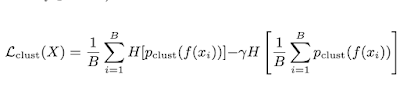## Sunday, September 27, 2020

### Implementing Clustering Loss For Deep Learning in Tensorflow 2.0

####The first term is the average entropy of the probabilities for each example using the softmax probabilities.
Each example xi in a batch is first embedded f(xi) and then pushed through the softmax. This term basically encourages assignment to a single cluster. The second term is the entropy of the average probability in each cluster over the complete batch. This is done so all clusters are used and the score discourages the assignment of all instances to the same batch. Below I posted my code for the loss function in Tensorflow.


class ClusteringLoss(Loss):

def __init__(self, gamma=1.0):
super().__init__()
self.gamma = gamma

def call(self, true, pred):
y  = pred + 1e-6
h1 = -1.0 * tf.reduce_sum(
tf.math.log(y) * y, axis=-1
)
H1 = tf.math.reduce_mean(h1)
total_y = tf.math.reduce_mean(y, axis=0)
H2 = -1 * tf.reduce_sum(
tf.math.log(total_y) * total_y
)
return H1 - self.gamma * H2



#### REFERENCES

 Jensen et. al.: "Coincidence, Categorization, and Consolidation: Learning to Recognize Sounds with Minimal Supervision", ICASSP 2020.